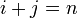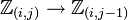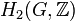# Group cohomology of Klein four-group

Jump to: navigation, search
This article gives specific information, namely, group cohomology, about a particular group, namely: Klein four-group.
View group cohomology of particular groups | View other specific information about Klein four-group

## Classifying space and corresponding chain complex

The classifying space of the Klein four-group is the product space$\mathbb{R}\mathbb{P}^\infty \times \mathbb{R}\mathbb{P}^\infty$, where$\mathbb{R}\mathbb{P}^\infty$ is infinite-dimensional real projective space.

A chain complex that can be used to compute the homology of this space is given as follows:

• The$n^{th}$ chain group is a sum of$n + 1$ copies of$\mathbb{Z}$, indexed by ordered pairs$(i,j)$ where$i + j = n$. In other words, the$n^{th}$ chain group is:$\mathbb{Z}_{(0,n)} \oplus \mathbb{Z}_{(1,n-1)} \oplus \dots \mathbb{Z}_{(n,0)}$

• The boundary map is given by adding up the following maps:
• The map$\mathbb{Z}_{(i,j)} \to \mathbb{Z}_{(i,j-1)}$ is multiplication by zero if$j$ is odd and is multiplication by two if$j$ is even.
• The map$\mathbb{Z}_{(i,j)} \to \mathbb{Z}_{(i-1,j)}$ is multiplication by zero if$i$ is odd and multiplication by two if$i$ is even.

## Homology groups

### Over the integers

The homology groups with coefficients in the ring of integers$\mathbb{Z}$ are given as follows:$H_p(\mathbb{Z}/2\mathbb{Z} \oplus \mathbb{Z}/2\mathbb{Z};\mathbb{Z}) = \lbrace\begin{array}{rl} (\mathbb{Z}/2\mathbb{Z})^{(p + 1)/2}, & p = 1,3,5,\dots \\ (\mathbb{Z}/2\mathbb{Z})^{p/2}, & p = 2,4,6,\dots \\ \mathbb{Z}, & p = 0 \\\end{array}$

These homology groups can be obtained from the knowledge of the homology groups of cyclic group:Z2 (see group cohomology of cyclic group:Z2) using the Kunneth formula. They can also be computed explicitly using the chain complex description above.

### Over an abelian group$M$

The homology groups with coefficients in an abelian group$M$ (which may be equipped with additional structure as a module over a ring$R$) are given as follows:

PLACEHOLDER FOR INFORMATION TO BE FILLED IN: [SHOW MORE]

## Cohomology groups and cohomology ring

### Groups over the integers

The cohomology groups with coefficients in the integers are given as below:$H^p(\mathbb{Z}/2\mathbb{Z} \oplus \mathbb{Z}/2\mathbb{Z};\mathbb{Z}) =$

## Second cohomology groups and extensions

### Schur multiplier

The Schur multiplier, defined as the second cohomoogy group for trivial group action$H^2(G,\mathbb{C}^\ast)$ and also as the second homology group$H_2(G,\mathbb{Z})$, is isomorphic to cyclic group:Z2.

See also the projective representation theory of Klein four-group.

### Second cohomology groups for trivial group action

Group acted upon Order Second part of GAP ID Second cohomology group for trivial group action Extensions Cohomology information
cyclic group:Z2 2 1 elementary abelian group:E8 elementary abelian group:E8, direct product of Z4 and Z2, quaternion group and dihedral group:D8 second cohomology group for trivial group action of V4 on Z2
cyclic group:Z4 4 1 elementary abelian group:E8 direct product of Z4 and V4, direct product of Z8 and Z2, central product of D8 and Z4, M16 second cohomology group for trivial group action of V4 on Z4
Klein four-group 4 2 elementary abelian group:E64 elementary abelian group:E16, direct product of Z4 and Z4, direct product of Z4 and V4, direct product of D8 and Z2, direct product of Q8 and Z2, SmallGroup(16,3), nontrivial semidirect product of Z4 and Z4 second cohomology group for trivial group action of V4 on V4
cyclic group:Z8 8 1 elementary abelian group:E8 direct product of Z8 and V4, direct product of Z16 and Z2, central product of D8 and Z8, M32 second cohomology group for trivial group action of V4 on Z8
direct product of Z4 and Z2 8 2  ?  ?  ?
elementary abelian group:E8 8 5  ?  ?  ?